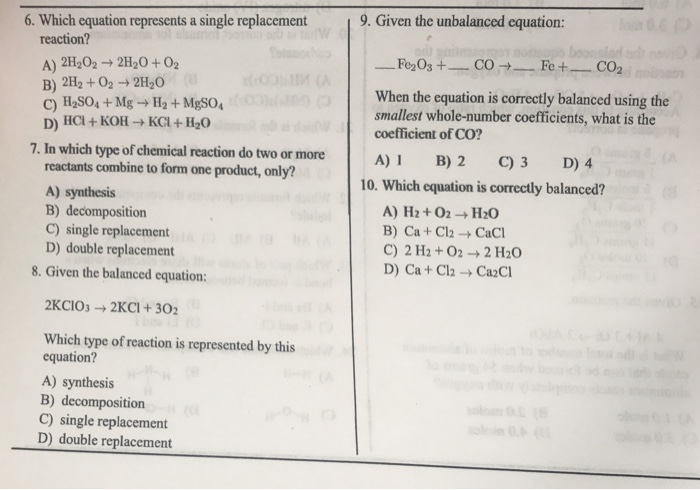# Question & Answer: Which equation represents a single replacement reaction? A) 2H_2O_2 rightarrow 2H_2O +…..Which equation represents a single replacement reaction? A) 2H_2O_2 rightarrow 2H_2O + O_2 B) 2H_2 + O_2 rightarrow 2H_2O C) H_2SO_4 + Mg rightarrow H_2 + MgSO_4 D) HCl + KOH rightarrow KCl + H_2O In which type of chemical reaction do two or more reactants combine to form one product, only? A) synthesis B) decomposition C) single replacement D) double replacement Given the balanced equation: 2KClO_3 rightarrow 2KCl + 3O_2 Which type of reaction is represented by this equation? A) synthesis B) decomposition C) single replacement D) double replacement Given the unbalanced equation: _____ Fe_2O_3 + _____ CO rightarrow _____ Fe + _____ CO_2 When the equation is correctly balanced using the smallest whole-number coefficients, what is the coefficient of CO? A) H_2 + O_2 rightarrow H_2O B) Ca + Cl_2 rightarrow CaCl C) 2 H_2 + O_2 rightarrow 2 H_2O D) Ca + Cl_2 rightarrow Ca_2Cl

1.single replacement reaction is one in which one elements is substittued for another elements in a compound. Accordingly, H2SO4+Mg——->MgSO4+H2, H2 is replaced by Mg. ( C is correct)

2. In synthesis reaction, two or more reactants combine to form products. In decomposition, the reactant decomposesto give more than one product. in single replacemt, one elements is substituted for another elements in a compound. In double displacement, one element of a compound gets substituted for another element of second compound.( A is correct)

Don't use plagiarized sources. Get Your Custom Essay on
Question & Answer: Which equation represents a single replacement reaction? A) 2H_2O_2 rightarrow 2H_2O +…..
GET AN ESSAY WRITTEN FOR YOU FROM AS LOW AS \$13/PAGE

3. Since KClO3 is giving two products, ir is decomposition reaction ( B is correct)

4. Fe2O3+(3/2)CO——>2Fe+(3/2)CO2 is the balanced reaction

2Fe2O3+3CO——–>4Fe+ 3CO2, the coefficient of CO is 3. ( C is the correct answer)

5. Balanced reaction, the no of atoms of each element on reactant side must be equal to no of atoms of the same element on the product side.

In H2+O2, there 2 oxygen atoms on reactant side and only one oxygen atom on product side.

Ca+Cl2, there are 2 chlorine atoms on reactant side and only one chlorine atom on CaCl2 side.

2H2+O2, there are 4 H atoms and two oxygen atoms while 2H2O also, there 4 H atoms and 2oxygen atoms. So balanced reaction. ( C is correct)

in Ca+Cl2, there is one Ca atoms while in Ca2Cl, there 2 Ca atoms. So not balanced.